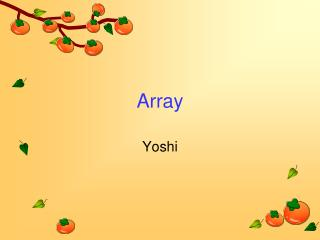DownloadDownload PresentationArray

# Array

Download Presentation## Array

- - - - - - - - - - - - - - - - - - - - - - - - - - - E N D - - - - - - - - - - - - - - - - - - - - - - - - - - -
##### Presentation Transcript

1. Array Yoshi

2. Definition • An array is a container object that holds a fixed number of values of a single type. • The length of an array is established when the array is created. After creation, its length is fixed.

3. Note • int[] anArray; • declares an array of integers • An array's type is written as type[] • [ ] are special symbols indicating that this variable holds an array.

4. Examples • The following are correct • double[] anArrayOfDoubles; • boolean[] anArrayOfBooleans; • char[] anArrayOfChars; • String[] anArrayOfStrings; • In fact, the following can also correct • Double anArrayOfDoubles[]; • But it is discouraged!!

5. Also objects • int[] anArray = newint; • Note that it uses the keyword new • anArray is a reference type variable to point to an array object • Try it • System.out.println( anArray.getClass() ); • System.out.println( anArray.length );

6. Initialization • int[] anArray = newint; • The content of an array will be initialized to the default values automatically • int[] anArray = {100, 200, 300, 400, 500, 600, 700, 800, 900, 1000}; • String[][] names = { {"Mr. ", "Mrs. ", "Ms. "}, {"Smith", "Jones"} }; • Note that names is a irregular array (not block style)

7. Irregular Array public class ArrayDemo { public static void main(String[] args) { int[][] array = new int[]; array = new int; array = new int; array = new int; System.out.println(array.length); //3 System.out.println(array.length); //8 System.out.println(array.length); //5 System.out.println(array.length); //10 } }

8. Copying Arrays • The System class has an arraycopy method that you can use to efficiently copy data from one array into another • public static void arraycopy(Object src, intsrcPos, Object dest, intdestPos, int length) • Of course, we can write a loop to do it by ourself

9. Example class ArrayCopyDemo { public static void main(String[] args) { char[] copyFrom = { 'd', 'e', 'c', 'a', 'f', 'f', 'e', 'i', 'n', 'a', 't', 'e', 'd' }; char[] copyTo = new char; System.arraycopy(copyFrom, 2, copyTo, 0, 7); System.out.println(new String(copyTo)); } }

10. For more array operations • java.util.Arrays • http://java.sun.com/javase/6/docs/api/java/util/Arrays.html • Such as sorting, binary search, etc

11. Pitfall • Student[] students = new Student; • System.out.println(students); • What’s the output?